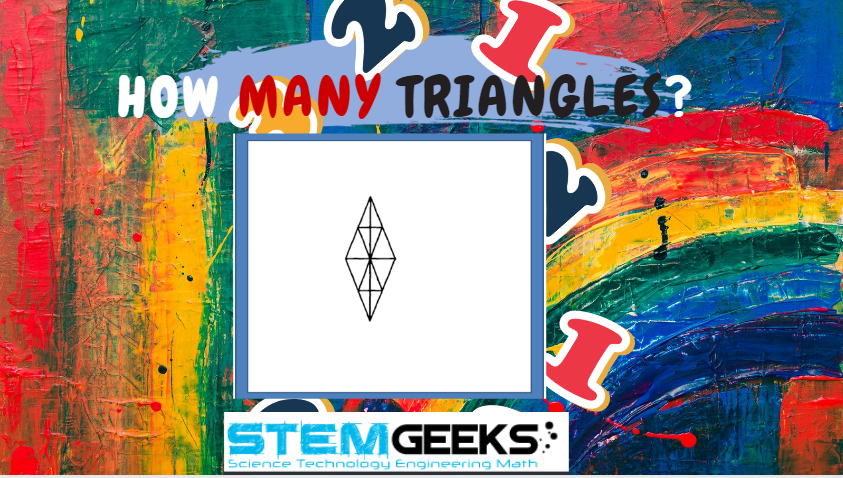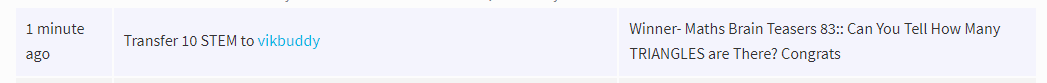# Maths Brain Teasers 84:: Can you Find the Missing Number???Hey All; @StemGeeks Mathematician;

Without further, ado, let's get to our puzzle for today:: Maths Brain Teasers 84:: Can you Find the Missing Number???Have a close look at the image given and see if you can decode the logic given in the image to complete the puzzle. Try finding out the right NUMBER that should replace the question mark? There is logic to resolve the puzzle; try finding out the logic and it should be resolved in seconds.. Guesswork isn't going to help...

With that, I'll leave you all with the Maths Brain Teasers 84:: Can you Find the Missing Number? Good luck solving the puzzle...

##### Maths Brain Teasers 83:: Can You Tell How Many TRIANGLES are There ?- Solved with ExplanationLet's have a look at the Step by Step instructions to Solve the Maths Brain Teasers 83:: Can You Tell How Many TRIANGLES are There?

If you carefully look at the Image in question; You would have noticed the following::

• First count the external Triangles given in the image i.e. the edges ONE
• Then move on counting the internal Triangles
• Then as a complete Triangle
• Followed by Triangles which are formed as part of half

Altogether there are 28 Triangles in the Image given.

Hence the answer to the Maths Brain Teasers 83:: Can You Tell How Many TRIANGLES are There? is :: 28 Triangles

### STEM token GiveAway

I'll be again doing a giveaway of STEM tokens to the lucky random winner with the correct answer. For the last contest, which was Maths Brain Teasers 83:: Can You Tell How Many TRIANGLES are There?.

We had 4 entries and I happy to see the engagement by everyone. At the same time, it's great to see the detailed answers provided by everyone as to how they arrived at the solution of the puzzle.. Well done guys.. Way to GO... Now the results part; out of all the entries, entry from @vikbuddy provided the right answer. So, he is the clear winner for the Maths Brain Teasers 83Congratulations @vikbuddy; WIN yourself 10 STEM tokens. You should be having the rewards in your STEM Wallet Soon.

### Math Quote for the Day::

Here is the motivation to solve the Maths Equation Puzzle?If you like my work, then please spread the Word.. that we do have the Math Brain Teasers competition here @StemGeeks platform. Reblog is much appreciated.

Best RegardsFind Me on the Other Social Media Platforms::PS:: All the Maths Brain Teasers; are made by me using the Pro Canva License Version

0
0
0.000I can't seem to figure things out so I guess I am guessing again today. I am going to guess the number 7.

0
0
0.000Is your number quiz guessing better than your football prediction guessing mate lol ;-)

0
0
0.000Probably not. I am going out on a wild guess because I spent 5-10 minutes trying to solve it or find a pattern. Since I didn't find it, I am moving on after giving out a random number.

0
0
0.000First count the external Triangles given in the image i.e. the edges ONE

Which external triangle? The external shape is a diamond or am I missing something mate? lol

Answer to today's puzzle is .....escaping me at the moment! I will I've it some thought and come back to you late!

0
0
0.000Tricky one for sure.

Only thing I can think of that sort of makes sense:

3 x (2) = 6
4x (2) = 8
1 x (9) = 9

Following that it's:

2 x (4.5) = 9

But the other numbers and the decimal don't make sense so doubt it's correct

Posted via proofofbrain.io

0
0
0.000Reason
Check the symmetry. Multiply the second digit with the number in bracket.

63 (2) 56 3 x 2 = 6
34 (2) 38 4 x 2 = 8
71 (9) 79 1 x 9 = 9
82 (?) 69 2 x 9/2 = 9